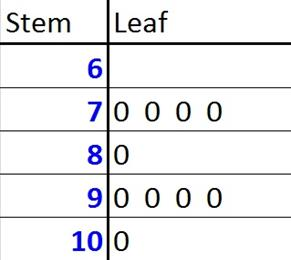© Ms. Garcia Math 7th The stem-and- leaf plot below shows test scores in Math. GR35 answersUse the stem and leaf plot to answer these questions. 1) Which of the following statements is true? I. The range is 40.  II. The mean is 82. A) I only B) II only B C) I and II D) Neither is true. Answer Name: ID: